## Python——变量和基本数据类型

2021/3/4 0:01:28 文章标签:

python对于初学者友好的地方就在于&#xff0c;没有一些固定的&#xff0c;格式化的东西需要写上&#xff0c;初学者可以开始自由的写点东西来开始学习python。 下面是我正在听歌的时候&#xff0c;随手写的打印歌词的代码。 song_name "光阴的故事" print(song_na…

python对于初学者友好的地方就在于，没有一些固定的，格式化的东西需要写上，初学者可以开始自由的写点东西来开始学习python。 下面是我正在听歌的时候，随手写的打印歌词的代码。

``````song_name = "光阴的故事"
print(song_name)
author = '作词：罗大佑'
print(author)
author = '作曲：罗大佑'
print(author)
author = '演唱：罗大佑'
print(author)
lrc_1 = "春天的花开秋天的风以及冬天的落阳"
print(lrc_1)
lrc_2 = "忧郁的青春年少的我曾经无知的这么想"
print(lrc_2)``````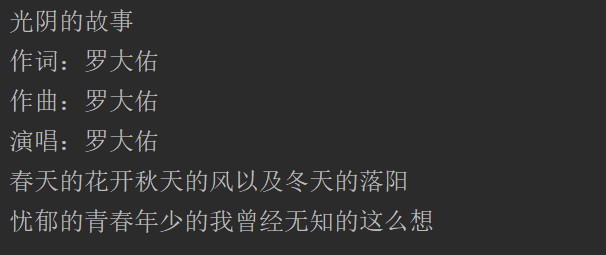``````姓名 = "张三"

print(姓名)
print(性别)
print(年龄)``````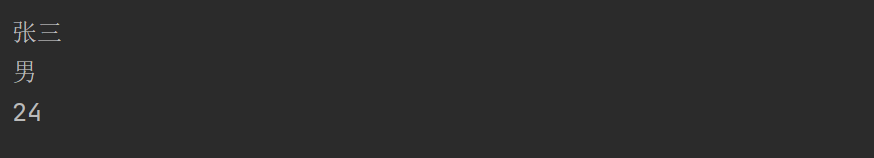``````message = '"Python is a good language！"'  # 这样就能很方便的打印双引号，而不需要使用转义字符
print(message)
message = "\"Python is a good language！\""  # 使用转义字符也能做的，就是麻烦了一些。
print(message)``````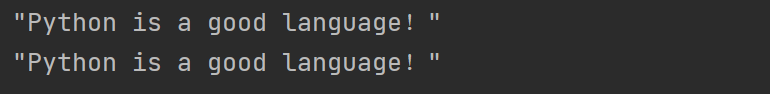## 字符串

``````name = input("Please input your name:")
print("Hello,",name)``````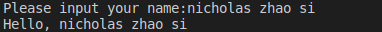``````name = input("Please input your name:")
print("Hello,",name.title())        #将用户输入的每一个单词的首字母变成大写。``````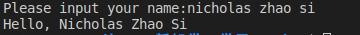``````name = input("Please input your name:")
print("Hello,",name.title())        #将用户输入的每一个单词的首字母变成大写。
print("name:",name)

name = name.title()     #改变name的值

print("name:",name)``````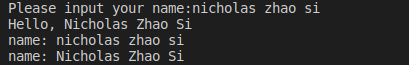upper()函数能够将所有字母转为大写；而lower()函数则相反，能够将所有字母转为小写。下面这个例子包含了简单的if-else语句，这和C语言的非常类似。

``````code = input("请输入验证码:X6sQ（不区分大小写）")
if code.upper() == "X6SQ":      #如果成立，打印OK
print("OK")
else:                           #否则，打印NO
print("NO")``````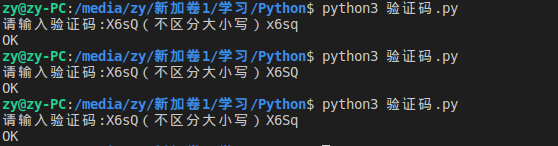``````code = input("请输入验证码:X6sQ（不区分大小写）")
if code.lower() == "x6sq":      #如果成立，打印OK
print("OK")
else:                           #否则，打印NO
print("NO")``````

``````name = input("请输入你的名字：")
out = F" 你好,{name}! "        #使用f字符串
print(out)        ``````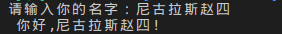python还内置了清空空白字符的函数，非常好用。例如下面所示：这个效果在终端下看起来是最明显的。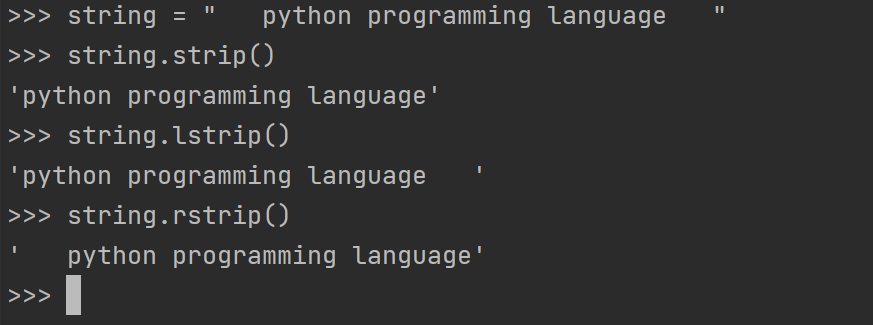``````name = "\tNicholas\tZhao\tSi\n"
print(name)
print(name.strip())
print(name.lstrip())
print(name.rstrip())``````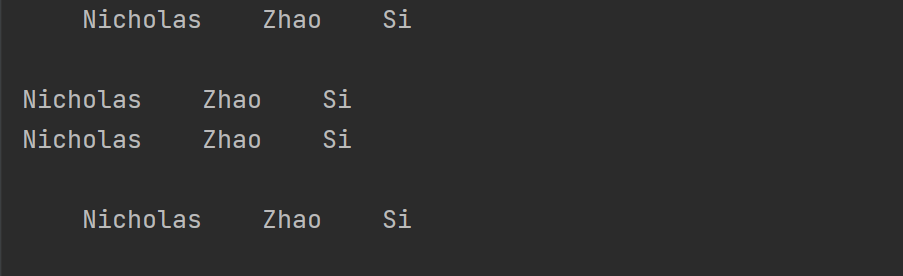``name = name.strip()``

## 数字类型

``````print(1+2)
print(1-2)
print(1*2)
print(1/2)
print(2/2)``````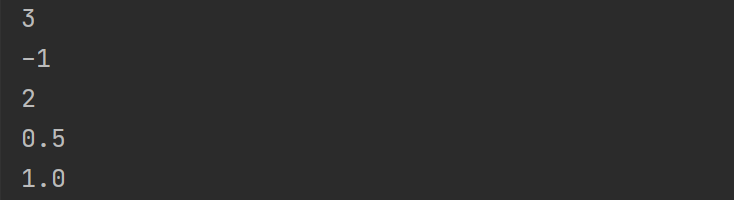``````print(2**10)
print(2*3.5)``````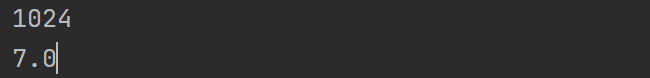python的浮点数同样也支持上面的所有操作。不过和其他语言的一样的是，浮点数总是不能够精确的表示。如下所示：

``````print(2.1**2.2)
print(3*0.1)``````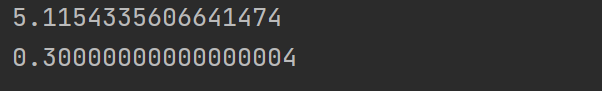python还非常人性化的提供了大数识别的操作。可以使用下划线给数字分组，来使得数据很容易的辨识出来。这对程序员真的太友好了。如下所示：

``````print(123_0000_0000)      # 中国人习惯4位一组
print(123_000_000)        # 外国人习惯3位一组``````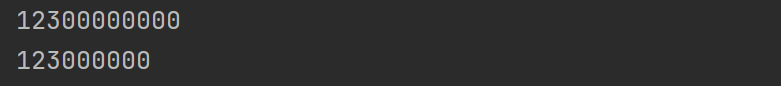python还支持同时给多个变量赋值。例如：

``````i, j, k = 1, 2, 3
print(i, j, k)``````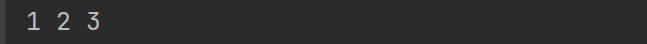``MAX_VALUE = 500``

``````print(32//4)
print(32/4)``````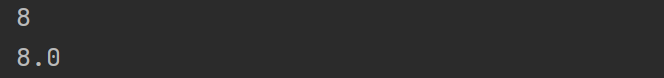``````"""

"""``````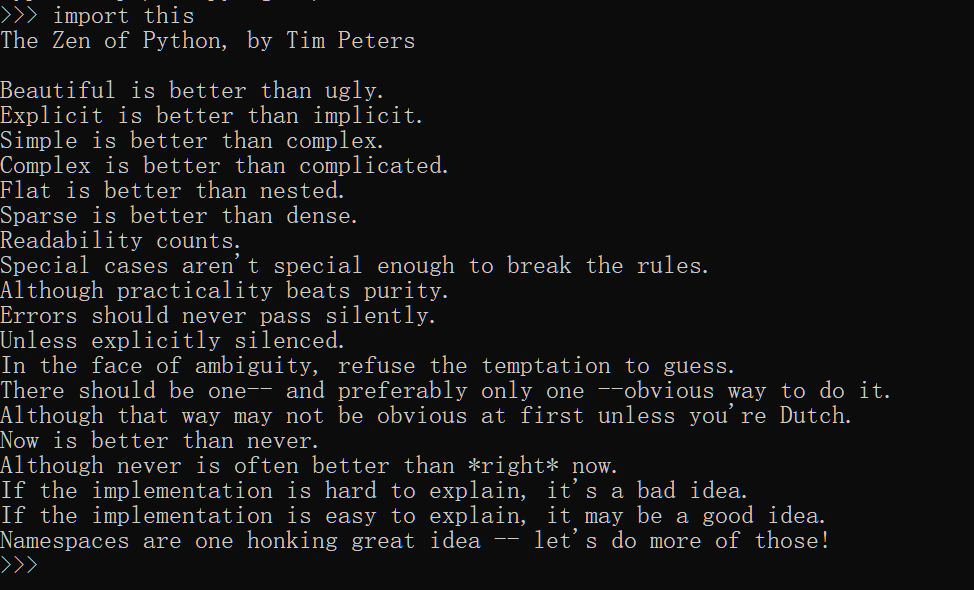暂无相关的数据...